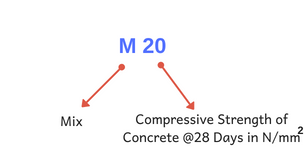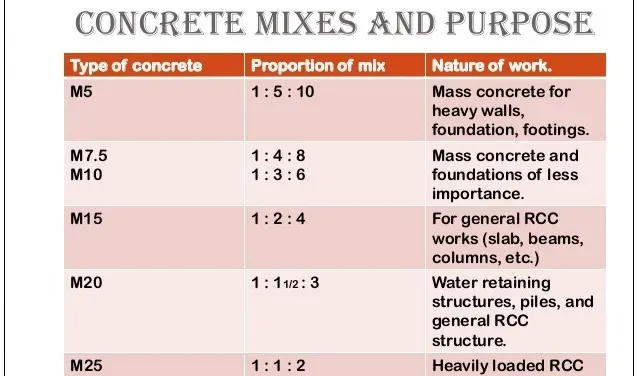Monday, September 25, 2023

# M20 Concrete Ratio (1:1.5:3) : Uses & Materials Required

M20 concrete ratio is 1:1.5:3. It indicates the cement:sand: aggregate ratio. It simply means that if 1 kg cement is used for making Concrete, you should use 1.5 kg sand and 3 kg aggregate. ( i.e. 1 part cement: 1.5 parts sand: 3 parts aggregate )

✔ In M20 concrete,“M” stands for Mix ratio of cement:sand: aggregate  &  “20 “Stands for Compressive Strength @ 28 Days in N/mm².

✔ M20 Concrete is nominal Concrete that can be skillfully prepared using Indian Standard IS 456:2000.

✔ Normally, about 38% of water is required for the complete hydration of Concrete.

As per Indian Standard IS 456:2000 for nominal mix ( i.e., M10, M15…, M25), the w/c ratio varies from 0.4 to 0.6.

For M20 Concrete, commonly w/c ratio of 0.45 to 0.50 is used.

### 1. Table1: M20 Concrete Ratio – Overview

 Code Indian Standard 10262(2009). Ratio 1:1.5:3 Compressive Strength 20 MPa { Note: MPa = N/mm²} Uses Used in RCC works like; a. Rigid Pavement Construction b. Used to construct structural members like slabs, beams, columns, etc. (for small to medium structures only. Not suitable for heavy structures or work). c. Water retaining structures, piles, etc. Grade Category Normal Grade ( Nominal Mix ) Required  Water Cement Ratio a. For high-quality concrete works; 0.45 w/c ratio is specified. b. Minimum water-cement ratio: 0.45 c. Maximum water-cement ratio: 0.50

### 2. M20 Concrete Ratio Uses

This concrete grade is commonly used for small to medium-scale constructions. Some common uses of the M20 Concrete Ratio are:✔ Used in the construction of water retaining structures.

✔ Used to construct piles and other general RCC structures.

✔ Used to build retaining walls, buildings, landscaping & architectural designs, etc.

### 3. Cement Sand Aggregate Calculation For 1 m³ M20 Concrete Ratio

Data per 1 m³ of M20 Concrete Ratio

 Material Volume Required (m3) Materials Required in kg Cement 0.28 403.2 Fine Aggregate 0.42 619.85 Coarse Aggregate 0.84 1359.82 Water 0.190 190 kg ( Average )

 Things To Know!! Density of = 1440 kg/m3 Density of = 1450-1500 Kg/m3 Density of Aggregate = 1450-1550 Kg/m3 Weight of Cement in 1 Bag = 50 Kg Volume of 1 Bag of Cement in Cubic meter = 0.03472 m3

Now, Taking

Grade of Concrete = M20 Concrete

Mix Ratio = 1:1.5:3

Total sum of ratio =1+1.5+3 =5.5

Wet volume of Concrete = 1 m3.

To get dry volume, wet volume increased by 54%

Dry volume of concrete = 1 + 0.54 = 1.54 m³

#### i. Cement Quantity Calculation

Quantity of cement = (Own Ratio/ Total sum of ratio) * Dry Volume

= ( 1/5.5 ) * 1.54

=  0.28 m³

Cement in kg = volume of cement * density of cement

= 0.28 * 1440

= 403.2 kg

#### ii. Sand Quantity Calculation

Quantity of = (Own Ratio/ Total sum of ratio) * Dry Volume

= ( 1.5/5.5 ) * 1.54

=  0.42 m³

#### iii. Aggregate Quantity Calculation

Quantity of = (Own Ratio/ Total sum of ratio) * Dry Volume

= ( 3/5.5 ) * 1.54

=  0.84 m³

#### iv. Water Calculation

For inferior-quality concrete: W/C ratio = 0.5

For superior-quality concrete: W/C ratio = 0.45

Minimum amount of water required per m³ = 0.45 * 403.2 = 181.44 kg

Maximum amount of water required per m³ = 0.5 * 403.2 = 201.6 kg

### 4. References

1. Indian Standard IS 456:2000

 Verified Article By Er. Madhu Krishna PoudelHe is a founder and lead author of Dream Civil International and his civil engineering research articles has been taken as source by world's top news and educational sites like USA Today, Time, The richest, Wikipedia, etc.
Latest Articles

Related Articles